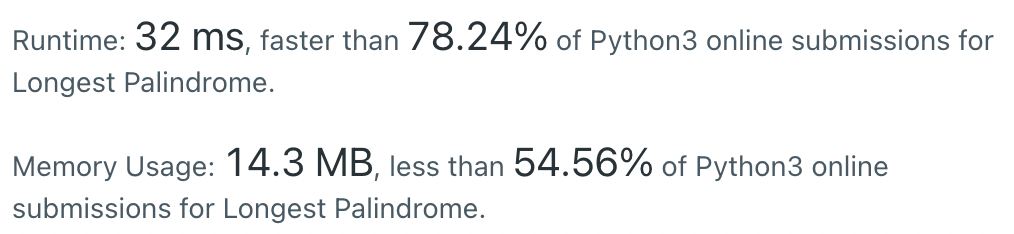#DAY 8
0

## 今日題目：409. Longest Palindrome

Given a string s which consists of lowercase or uppercase letters, return the length of the longest palindrome that can be built with those letters.
Letters are case sensitive, for example, "Aa" is not considered a palindrome here.

Example 1:
Input: s = "abccccdd"
Output: 7
Explanation:
One longest palindrome that can be built is "dccaccd", whose length is 7.

Example 2:
Input: s = "a"
Output: 1

### 解題技巧

#### 1. str.count(sub, start= 0,end=len(string))

str = 'banana pie banana'
str.count('a',2)
->5

#### 2. collections.Counter()

• c = Counter(str2)
->Counter({'a': 3, 'n': 2, 'b': 1, 's': 1})

• Counter.items()->['a': 3, 'n': 2, 'b': 1, 's': 1]

• Counter.values()->[3,2,1,1]

• Counter.keys()->['a','n','b','s']

### My solution

``````class Solution:
def longestPalindrome(self, s: str) -> int:
n = len(s)
c = collections.Counter(s)

ans = 0
odd = 0

for v in c.values():
ans += int(v/2)*2

if ans%2 ==0 and v%2==1:
ans +=1
return ans
``````

### Result